# The Taylor series of the Gaussian kernel

## Transcription

The Taylor series of the Gaussian kernel
```THE TAYLOR SERIES OF THE GAUSSIAN KERNEL
L. ESCAURIAZA
”From some people one can learn more than mathematics”
Abstract. We describe a formula for the Taylor series expansion of the Gaussian kernel around the origin of Rn × R.
1. Introduction
The explicit formulae for the power series expansion at the origin of the fundamental solution of the Laplace operator in Rn , n ≥ 2, are well known. In particular,
when
1
Γ(x, y) =
|x − y|2−n ,
ωn (2 − n)
ωn is the surface measure of the unit sphere Sn−1 in Rn and n > 2, or when
1
Γ(x, y) =
log |x − y|
2π
and n = 2, the following hold:
Z
(1.1)
ϕ(x) =
Γ(x, y)4ϕ(y) dy , when ϕ ∈ C0∞ (Rn ) ,
Rn
(1.2)
Γ(x, y) =
+∞
X
k=0
(1.3)
|x|k
Zk (x0 · y 0 ) , when 0 ≤ |x| < |y| ,
|y|k+n−2
(
(k)
− 1 Z 0 (y 0 )
Zk (x · y ) = 1 2k+n−2 x
2π log |y|
0
0
, when 2k + n − 2 > 0 ,
, when 2k + n − 2 = 0 .
(k)
Here, x0 = x/|x| and Zx0 (y) is the zonal harmonic of degree k, i.e., the kernel of
the projection operator of L2 (S n−1 ) onto the spherical harmonics of degree k ≥ 0.
We recall that the the spherical harmonics of degree k ≥ 0 are the eigenfunctions of
the spherical Laplacian on Sn−1 and corresponding to the eigenvalue, k(k + n − 2).
See [8, (2.17)] for (1.1) and [11, Chapter IV] for (1.2) and (1.3). 1
For fixed z 0 in S n−1 and k ≥ 0, the function
Ek (x) = |x|k Zk (x0 · z 0 )
1991 Mathematics Subject Classification. Primary: 35K05; Secondary: 35C10.
Key words and phrases. Gaussian kernel.
The author is supported by MEC grant MTM2004-03029 and by the UPV/EHU grant 9/UPV
00127.310-15969/2004.
1A simple way to derive (1.2) and (1.3) is to solve, 4u = f in Rn , via the method of separation
of variables in spherical coordinates and then, to compare the solution, which the latter method
yields, with the one obtained via the convolution with the fundamental solution.
1
2
L. ESCAURIAZA
is a homogeneous harmonic polynomial of degree k, i.e.,
4Ek = 0 and Ek (λx) = λk Ek (x) ,
when λ ≥ 0 and x is in Rn . The function,
|y|2−n−k Zk (z 0 · y 0 )
is harmonic in Rn \ {0} and is homogeneous of degree, 2 − k − n. In fact, it is is
the Kelvin transformation of Ek . Here recall that the Kelvin transformation of a
function u is the function
v(x) = |x|2−n u(x/|x|2 ) ,
and that
4v(x) = |x|−n−2 4u(x/|x|2 ) .
Also,
ϕ=
+∞
X
and kϕk2L2 (Sn−1 ) =
Zk (ϕ)
k=0
+∞
X
kZk (ϕ)k2L2 (Sn−1 ) ,
k=0
when ϕ is in C ∞ (Sn−1 ) and
Z
Zk (ϕ) =
Sn−1
(k)
Zx0 (y 0 )ϕ(y 0 ) dy 0 , when k ≥ 0 .
Moreover, the formula (1.2) is the Taylor series expansion of Γ(x, y) around
x = 0, for each fixed y 6= 0 in Rn . (See [11, Chapter IV]).
The Taylor series (1.2) contains relevant information, which has had important
applications and among others, it has shown to be useful to obtain estimates leading
to sharp results of strong and weak unique continuation for elliptic operators on
Rn . This can be seen in ,  and .
The Gaussian kernel
(
−n/2 −|x−y|2 /4(t−s)
(4π(t − s))
e
, when s < t ,
G(x, t, y, s) =
0
, when s > t ,
is the fundamental solution of the heat operator in Rn+1 , i.e.
Z t Z
f (x, t) = −
G(x, t, y, s)(4f − ∂s f ) dyds , when f ∈ C0∞ Rn+1 .
−∞
Rn
As far as the author knows, it seems that nobody has written down and publish
an explicit formula for the Taylor series expansion of G(x, t, y, s) around the origin
of Rn+1 , when (y, s) in Rn+1 , s < 0, is fixed. The purpose of this note is to fill in
this gap.
To simplify the notation, we choose to give the formula for the Taylor series
expansion of the fundamental solution of the backward heat equation,
(
−n/2 −|x−y|2 /4(s−t)
(4π(s − t))
e
, when t < s ,
(1.4)
Gb (x, t, y, s) =
0
, when t > s ,
when s is positive and (y, s) in Rn+1 is fixed. The Taylor series for the Gaussian
kernel follows from the identity
G(x, t, y, s) = Gb (x, −t, y, −s) .
THE TAYLOR SERIES OF THE GAUSSIAN KERNEL
3
The Hermite functions, hk , are defined as
√ − 1
2
2
dk hk (x) = 2k k! π 2 (−1)k ex /2 k e−x
, k≥0, x∈R
dx
2
and hk = Hk (x)e−x /2 , where Hk is a Hermite polynomial of degree k.
The Hermite functions on Rn , φα , α = (α1 , . . . , αn ) in Nn , are the product of
the one-dimensional Hermite functions hαj , j = 1, . . . , n
φα (x) =
n
Y
hαj (xj ) .
j=1
They form a complete orthonormal system in L2 (Rn ) and if, H = 4 − |x|2 , is the
Hermite operator, Hφα = − (2 |α| + n) φα , where |α| = α1 + · · · + αn . The kernel
X
Φk (x, y) =
φα (x)φα (y)
|α|=k
is the kernel of the projection operator of L2 (Rn ) onto the Hermite functions of
degree, k ≥ 0, and when ϕ ∈ C0∞ (Rn ),
ϕ=
+∞
X
, kϕk2L2 (Rn ) =
Pk (ϕ)
k=0
+∞
X
kPk (ϕ)k2L2 (Rn )
k=0
and where
Z
Φk (x, y)ϕ(y) dy , k ≥ 0 .
Pk (ϕ) =
Rn
The reader can find the proofs of the latter results in [13, Chapter 1].
What seems to be the counterpart of the Kelvin transformation in the parabolic
setting is the Appell transformation v of a function u (, [2, pp. 282]):
v(x, t) = |t|−n/2 e−|x|
2
/4t
u(x/t, 1/t) ,
which verifies
4v − ∂t v = |t|−2−n/2 e−|x|
2
/4t
(4u + ∂t u)(x/t, 1/t)
and the Appell transformation maps backward caloric functions into forward caloric
functions.
A calculation shows that
√
2
Qα (x, t) = tk/2 φα (x/2 t)e|x| /8t , α ∈ Nn , |α| = k ,
is backward caloric and in fact, it is a backward caloric polynomial in the (x, t)variables, which is homogeneous of degree k = |α| in the parabolic sense, i.e.,
4Qα + ∂t Qα = 0 in Rn+1 and Qα (λx, λ2 t) = λk Qα (x, t) ,
when λ ≥ 0 and (x, t) is in Rn+1 (The later follows because a Hermite polynomial
Hk is an even function, when k is even and an odd function, when k is odd). At the
same time and in analogy with the what happens with the Kelvin transformation
of the harmonic function, |x|k Zk (z 0 · x0 ), the function
√
2
s−(k+n)/2 φα (y/2 s)e−|y| /8s ,
is forward caloric and is the Appell transformation of Qα .
4
L. ESCAURIAZA
Having gathered all this data, it is possible to describe and write down the Taylor
series expansion of the backward Gaussian kernel (1.4) at the origin of Rn+1 . We
do it in the following theorem:
Theorem 1. The following identity holds, when t < s, s > 0, and x, y are in Rn
!
+∞
X
√
√
2
k/2
−n/2 |x|2 /8t
(1.5) Gb (x, t, y, s) = (4s)
e
(t/s) Φk (x/2 t, y/2 s) e−|y| /8s .
k=0
The proof of Theorem 1 is given in section 2 and it follows from a well known
identity: the generating formula for the kernels, Φk (See (2.1) below).
The commentaries in [12, pp. 582–583], which are made with the purpose to
explain the reader a simple approach to prove the identity (2.1) and in particular,
the reference [6, pp. 335–336], show that Theorem 1 was probably already known
to some authors, though not explicitly written down and published. In fact, the
approach suggested in [12, pp. 582–583] to prove the identity (2.1), follows precisely
the inverse path of the one we follow in section 2 to prove Theorem 1. Thus,
Theorem 1 was probably known by W. Feller and E.M. Stein.
In the same way as the formula for the Taylor series of the fundamental solution
of the Laplace operator has been useful to derive results of unique continuation for
elliptic operators, the formula in Theorem 1 is what, in a certain sense, is behind
the positive results of unique continuation for parabolic equations in , ,  and
.
The argument is section 2 gives a clue of how to proceed to find the Taylor
series expansion of the fundamental solution of the Schr¨odinger operator, 4 + i∂t ,
around the origin of Rn+1 and the corresponding building pieces of the solutions
of the Schr¨
odinger equation: “the Schr¨
odinger homogeneous polynomials of degree
k ≥ 0”.
2. Proof of Theorem 1
Proof. Recall the generating formula for the projection kernels, Φk 
(2.1)
+∞
X
− n − 1 1+ξ2 |x|2 +|y|2 )+ 2ξxy2
n
1−ξ
, when |ξ| < 1 , ξ ∈ C .
Φk (x, y)ξ k = π − 2 1 − ξ 2 2 e 2 1−ξ2 (
k=0
p
√
√
Replace x by x/2 t, y by y/2 s and take ξ = t/s in (2.1), when 0 ≤ t < s. It
gives
(2.2)
+∞
X
√
xy
s+t
2
2
√
n
−n
k/2
s−n/2
(t/s) Φk (x/2 t, y/2 s) = π − 2 (s − t) 2 e− s−t (|x| /8t+|y| /8s)+ 2(s−t) .
k=0
Then, multiply (2.2) by 4−n/2 e|x|
(4s)−n/2 e|x|
2
/8t
2
/8t−|y|2 /8s
to get that the identity
!
+∞
X
√
√
2
k/2
(t/s) Φk (x/2 t, y/2 s) e−|y| /8s
k=0
−n
2
= (4π(s − t))
e−|x−y|
holds, when 0 ≤ t < s, s > 0 and x, y are in Rn , and Theorem 1 follows.
2
/4(s−t)
THE TAYLOR SERIES OF THE GAUSSIAN KERNEL
5
References
∂ 2 z/∂ 2 x
 P. Appell, Sur l’´
equation
− ∂z/∂y = 0 et la th´
eorie du chaleur, J. Math. Pures.
Appl. (4) 8 (1892), 187–216.
 J.L. Doob, Classical potential theory and its probabilistic counterpart, Springer-Verlag NewYork Berlin, Heildeberg Tokyo, 1984.
 L. Escauriaza, Carleman inequalities and the heat operator, Duke Math. J. 104, n.1 (2000),
113-127.
 L. Escauriaza, F.J. Fern´
andez, Unique continuation for parabolic operators, Ark. Mat. 41
(2003), 35–60.
 L. Escauriaza, L. Vega, Carleman inequalities and the heat operator II, Indiana U. Math. J.
50, n.3 (2001), 1149–1169.
 W. Feller, An introduction to probability theory and its applications, Vol. II, Wiley.
 F.J. Fern´
andez, Unique continuation for parabolic operators II, Comm. Part. Diff. Equat. 28
n. 9 & 10 (2003), 1597–1604.
 D. Gilbarg, N.S. Trudinger, Elliptic partial differential equations of second order, SpringerVerlag Berlin, Heildeberg, New-York, Tokyo, 1983.
 D. Jerison, C.E. Kenig, Unique continuation and absence of positive eigenvalues for
Schr¨
odinger operators, Annals of Math. 121 (1985), 463–488.
 H. Koch, D. Tataru, Carleman estimates and unique continuation for second order elliptic
equations with nonsmooth coefficients, Comm. Pure Appl. Math.54 n. 3 (2001), 339–360.
 E.M. Stein, Introduction to Fourier analysis on euclidean spaces, Princeton, New Jersey.
Princeton University Press, 1975.

, Harmonic Analysis: Real-variable Methods, Orthogonality and Oscillatory Integrals,
Princeton University Press, 1993.
 S. Thangavelu, Lectures on Hermite and Laguerre expansions, Princeton Univ. Press, Princeton, New Jersey, 1993.
 T. Wolff, Unique continuation for |4u| ≤ V |∇u| and related problems, Rev. Mat. Iberoamericana 6 (1990), 155–200.
(L. Escauriaza) Universidad del Pa´ıs Vasco / Euskal Herriko Unibertsitatea, Departamento de Matematicas, Apartado 644, 48080 Bilbao, Spain.
E-mail address: [email protected]
```

### Fiter: Greedy Bloggers for a Picky Audience Avner May, AugustinMore information

### MATH 3078/3978 — Partial Differential Equations and Waves — Assignment 2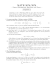More information

### FULL BIO-DES 2015.indd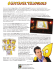More information

### 2013 Competitive Grantmaking Evaluation Criteria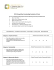More information

### Field-trips Field-trips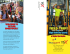More information

### Taylor Eblast 12_20_10More information

### Desoutter tools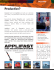More information

### Waterchase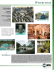More information

### IT`S TIME TO PROVE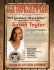More information

### Ebo Taylor - My Love sales notes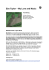More information

### Ch8 - Department of Engineering and Physics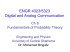More information

### arXiv:1501.03050v1 [math.AP] 13 Jan 2015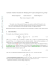More information